Like   Tweet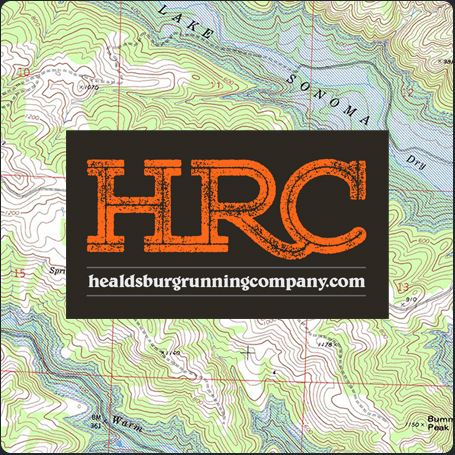table div table+table+table div table{width:100%;padding:0}table div table+table+table div table img{width:96.23%;padding:0;float:none}table div table+table+table div table td{width:100%;padding:0 1.88% 18px}/* styles */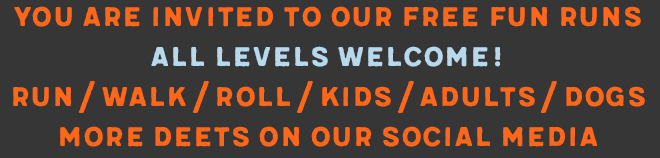table div table+table+table+table+table div table{width:100%;padding:0}table div table+table+table+table+table div table img{width:96.23%;padding:0;float:none}table div table+table+table+table+table div table td{width:100%;padding:0 1.88% 18px}/* styles */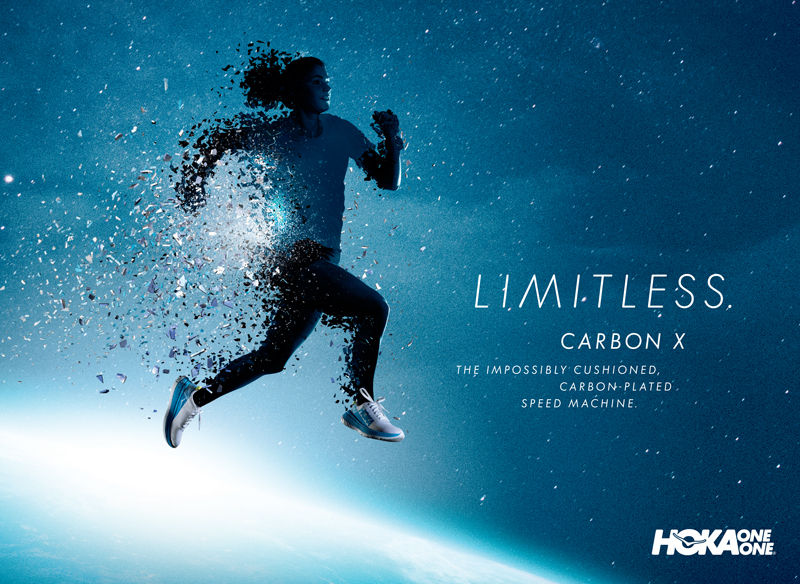/* styles */ Thursday May 9, 2019 at 6pm Pizza & Pints HOKA ONE ONE® & Iron Man Shake-out Run with Finish at Pizzando River Running on Bailhache Avenue with the Newest HOKA's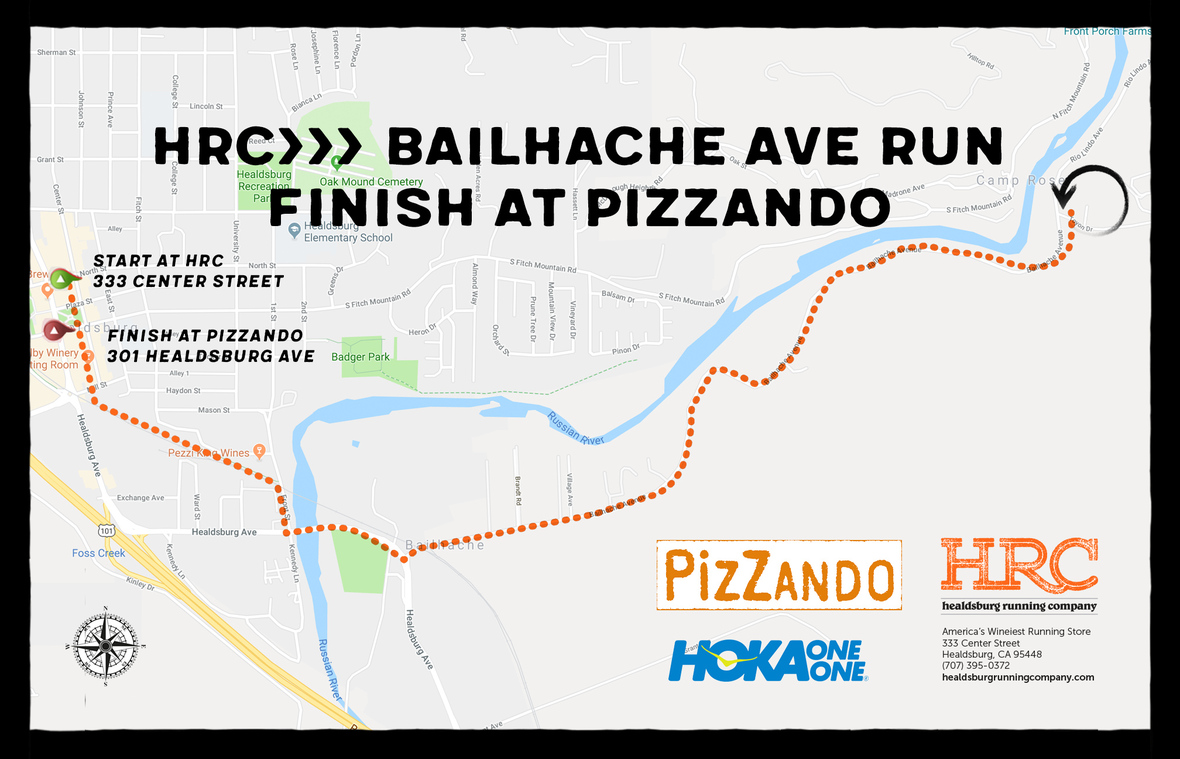table div table+table+table+table+table+table+table+table+table div table{width:100%;padding:0}table div table+table+table+table+table+table+table+table+table div table img{width:96.23%;padding:0;float:none}table div table+table+table+table+table+table+table+table+table div table td{width:100%;padding:0 1.88% 18px}/* styles */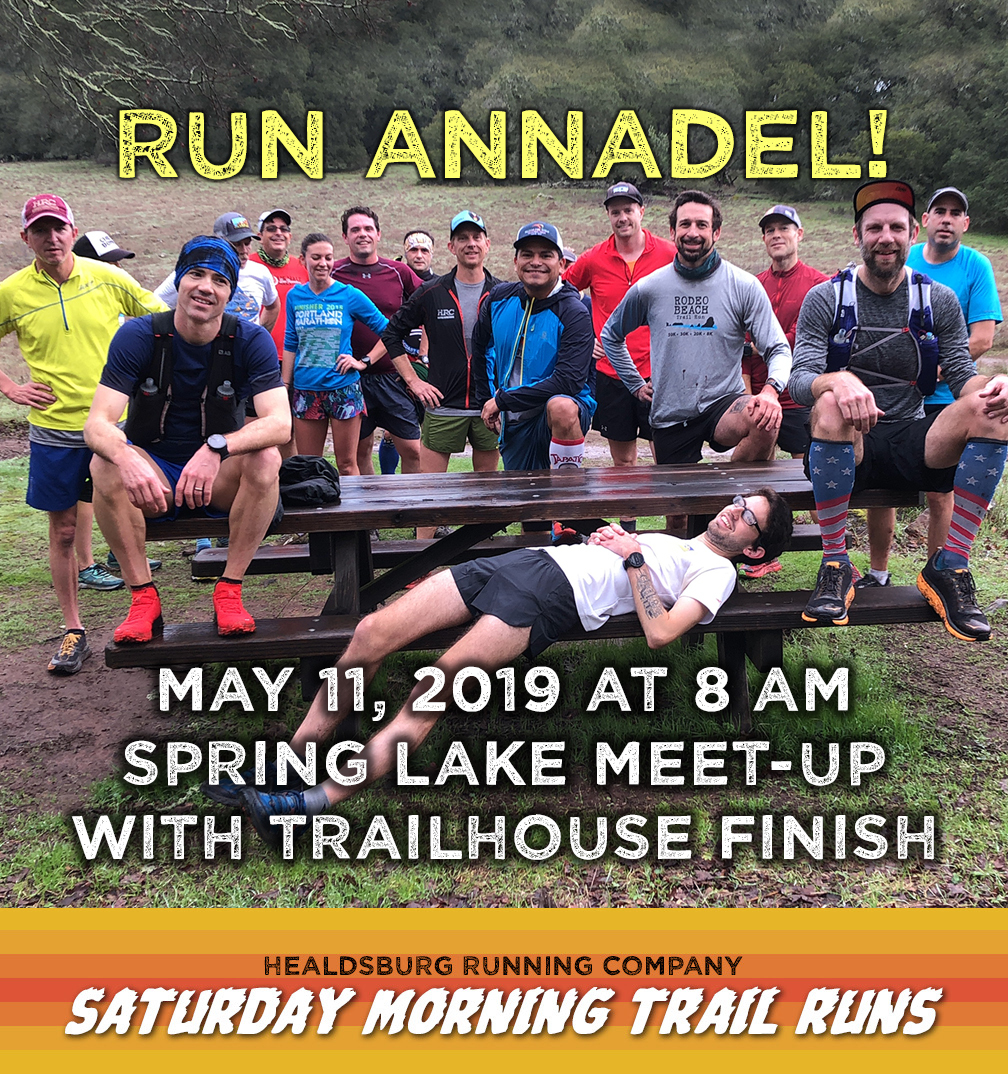/* styles */ Meeting at Spring Lake and Running Lake Ilsanjo with HOKA ONE ONE® Shoes and Roka Goggles! Dualathon Style – 6 Mile Trail Run and 1 Mile Swim or 10 Mile Trail Run – Finish at Trailhouse for Free Beers for the Duathletes.
 table div table+table+table+table+table+table+table+table+table+table+table+table div table{width:100%;padding:0}table div table+table+table+table+table+table+table+table+table+table+table+table div table img{width:96.23%;padding:0;float:none}table div table+table+table+table+table+table+table+table+table+table+table+table div table td{width:100%;padding:0 1.88% 18px}/* styles */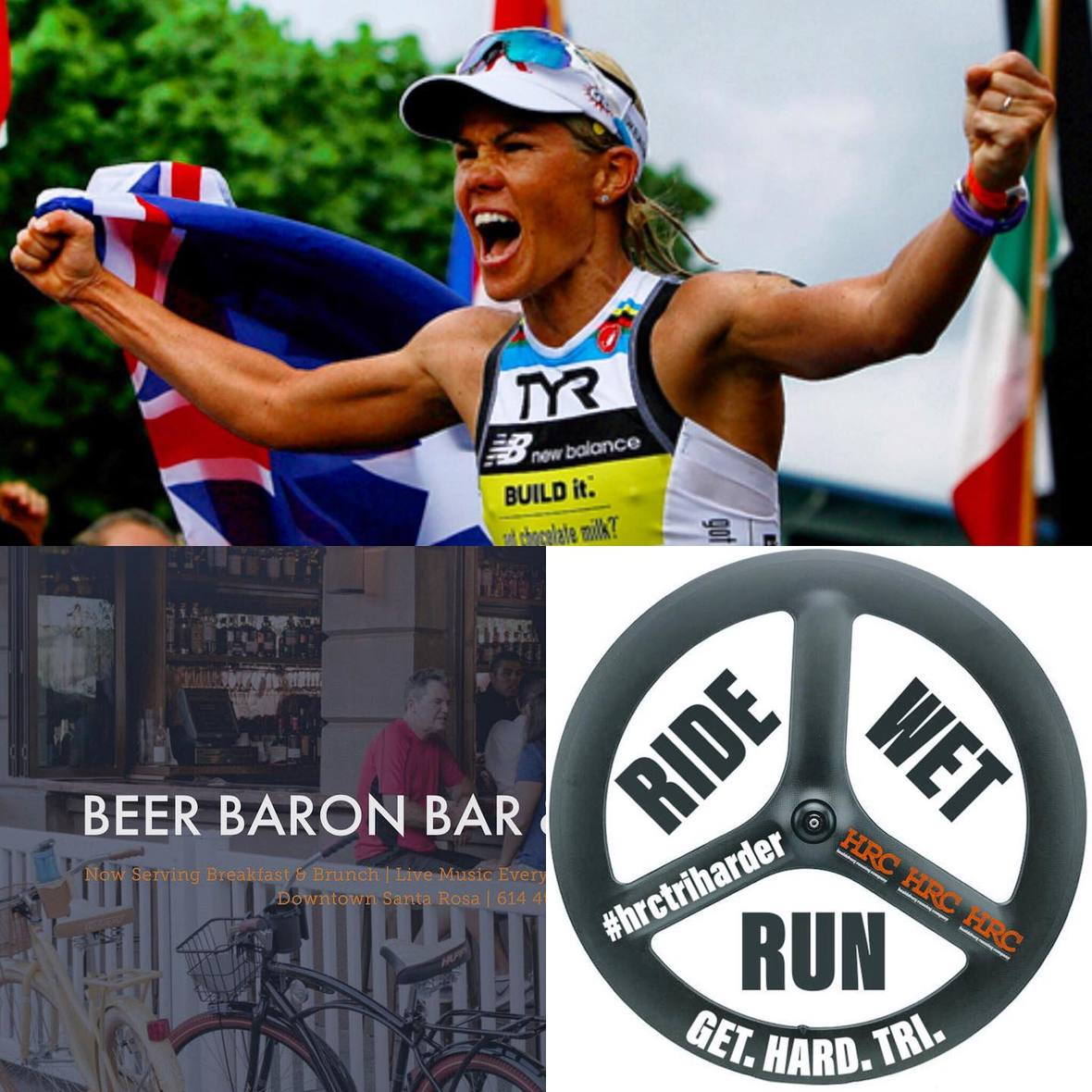/* styles */ Saturday May 11 - IRONMAN Back of the Pack Celebration! 7 pm till? Beers, Food, IRONMAN and Santa Rosa! Look for the VIP Outside Tables Close to the Finish! @ironmantri #hrcrunco #hrctriharder
 table div table+table+table+table+table+table+table+table+table+table+table+table+table+table+table div table{width:100%;padding:0}table div table+table+table+table+table+table+table+table+table+table+table+table+table+table+table div table img{width:96.23%;padding:0;float:none}table div table+table+table+table+table+table+table+table+table+table+table+table+table+table+table div table td{width:100%;padding:0 1.88% 18px}/* styles */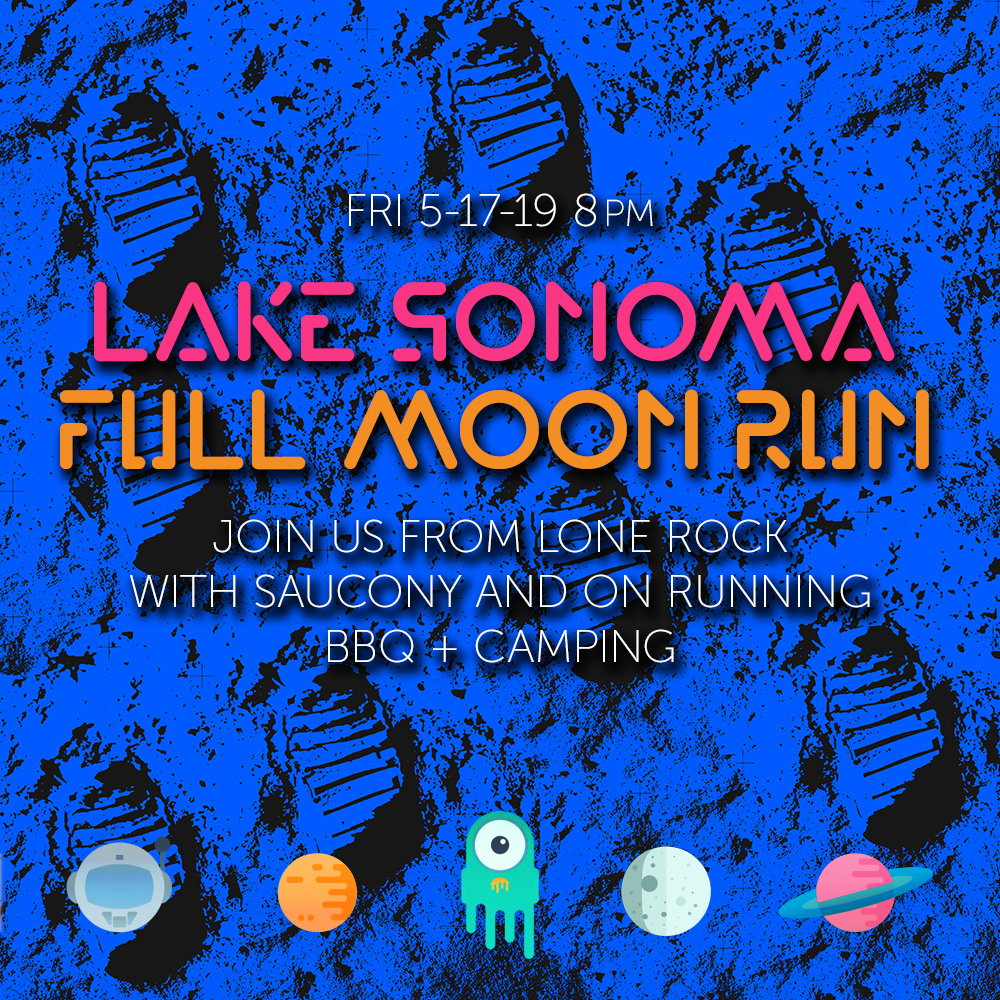table div table+table+table+table+table+table+table+table+table+table+table+table+table+table+table+table+table div table{width:100%;padding:0}table div table+table+table+table+table+table+table+table+table+table+table+table+table+table+table+table+table div table img{width:96.23%;padding:0;float:none}table div table+table+table+table+table+table+table+table+table+table+table+table+table+table+table+table+table div table td{width:100%;padding:0 1.88% 18px}/* styles */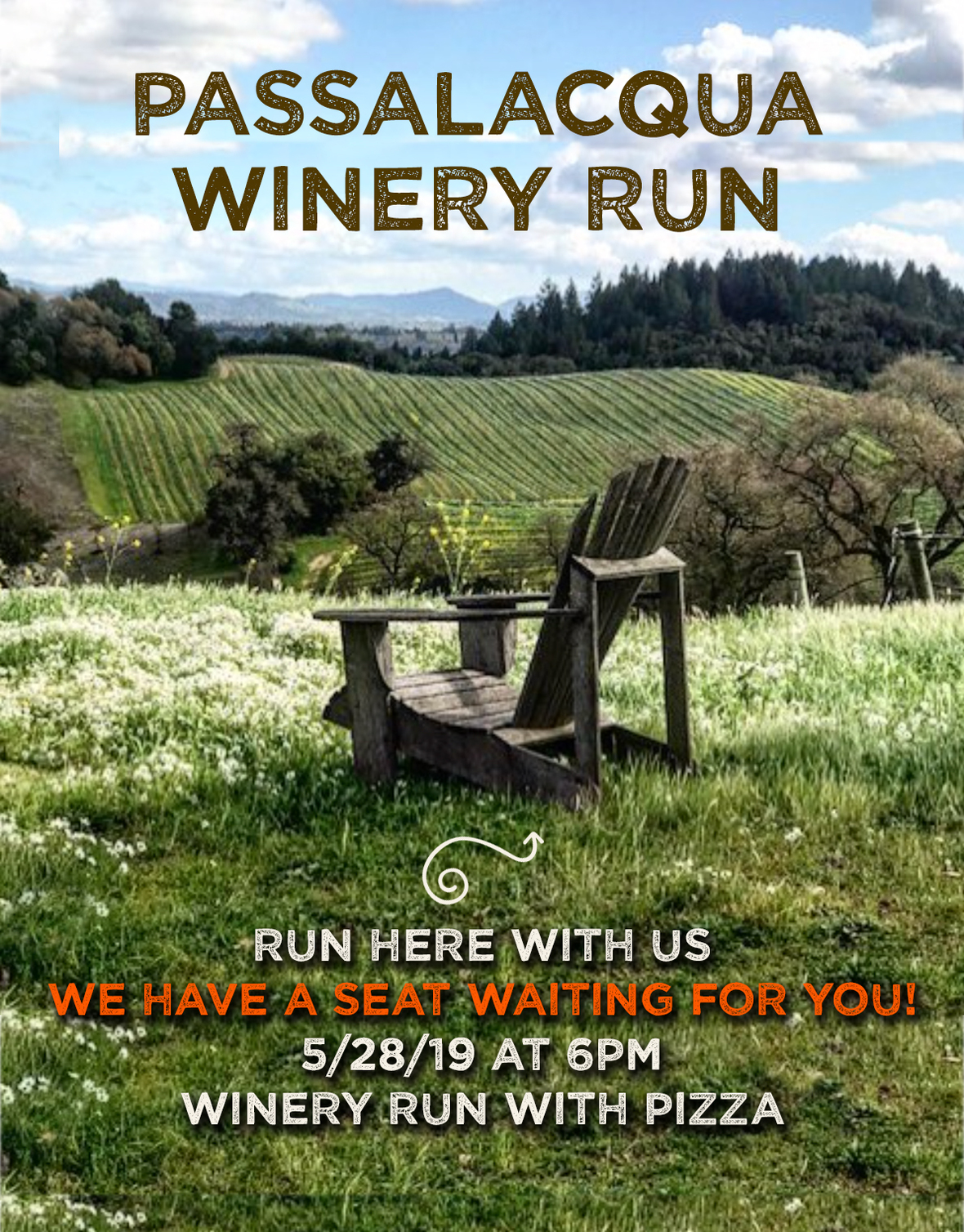table div table+table+table+table+table+table+table+table+table+table+table+table+table+table+table+table+table+table+table div table{width:100%;padding:0}table div table+table+table+table+table+table+table+table+table+table+table+table+table+table+table+table+table+table+table div table img{width:96.23%;padding:0;float:none}table div table+table+table+table+table+table+table+table+table+table+table+table+table+table+table+table+table+table+table div table td{width:100%;padding:0 1.88% 18px}/* styles *//* styles */ Healdsburg Running Company 333 Center Street, Healdsburg, CA (707) 395-0372 Mon-Sat 10 am – 7 pm Sunday 11 am – 5 pm Running shoes (trail & road), apparel, nutrition, runs, races, gear, ambassadors and more... More Deets on Our Social Media